Radians are another way to measure angles (as opposed to degrees.) Radians are used extensively in calculus and factor more easily into advanced calculations.

Radians use the idea of a unit circle. One radian is approximately 57.3° and results when the arc length is equal to the radius of the circle. One radian is shown here: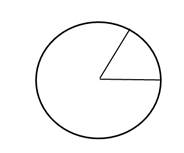Because the circumference of a circle equals 2∏r, the entire circle (360°) contains 2∏ radians. That means that half a circle (180°) equals ∏ radians. See the picture here: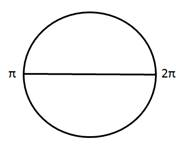Radians are often listed with ∏ because that produces an exact answer as opposed to an approximation. We can further divide our unit circle into divisions. For example, if we divide ∏ into thirds, we get the following: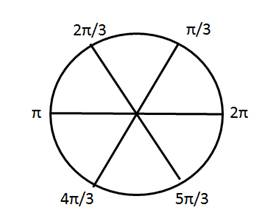Here it is divided in fourths.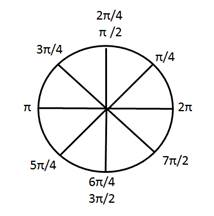And we could in this same manner divide it as many times as we want.

Practice: Match the location on the left with the radian measure on the right.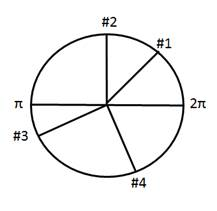Answers: 1) B      2) A      3) F     4) E

 Related Links: Math Fractions Factors